The OpenFOAM Foundation
perfectGas< Specie > Class Template Reference

Perfect gas equation of state. More...

Inheritance diagram for perfectGas< Specie >: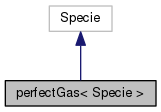[legend]
Collaboration diagram for perfectGas< Specie >:[legend]

## Public Member Functions

perfectGas (const Specie &sp)
Construct from components. More...

perfectGas (const dictionary &dict)
Construct from dictionary. More...

perfectGas (const word &name, const perfectGas &)
Construct as named copy. More...

autoPtr< perfectGasclone () const
Construct and return a clone. More...

scalar rho (scalar p, scalar T) const
Return density [kg/m^3]. More...

scalar H (const scalar p, const scalar T) const
Return enthalpy departure [J/kg]. More...

scalar Cp (scalar p, scalar T) const
Return Cp departure [J/(kg K]. More...

scalar S (const scalar p, const scalar T) const
Return entropy [J/(kg K)]. More...

scalar psi (scalar p, scalar T) const
Return compressibility rho/p [s^2/m^2]. More...

scalar Z (scalar p, scalar T) const
Return compression factor []. More...

scalar CpMCv (scalar p, scalar T) const
Return (Cp - Cv) [J/(kg K]. More...

void write (Ostream &os) const
Write to Ostream. More...

void operator+= (const perfectGas &)

void operator*= (const scalar)

## Static Public Member Functions

static autoPtr< perfectGasNew (const dictionary &dict)

static word typeName ()
Return the instantiated type name. More...

## Static Public Attributes

static const bool incompressible = false
Is the equation of state is incompressible i.e. rho != f(p) More...

static const bool isochoric = false
Is the equation of state is isochoric i.e. rho = const. More...

## Friends

perfectGas operator+ (const perfectGas &, const perfectGas &)

perfectGas operator* (const scalar s, const perfectGas &)

perfectGas operator== (const perfectGas &, const perfectGas &)

Ostreamoperator (Ostream &, const perfectGas &)

## Detailed Description

### template<class Specie> class Foam::perfectGas< Specie >

Perfect gas equation of state.

Source files

Definition at line 47 of file perfectGas.H.

## ◆ perfectGas() [1/3]

 perfectGas ( const Specie & sp )
inline

Construct from components.

Definition at line 31 of file perfectGasI.H.

References Foam::name().

Here is the call graph for this function: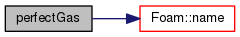## ◆ perfectGas() [2/3]

 perfectGas ( const dictionary & dict )

Construct from dictionary.

Definition at line 32 of file perfectGas.C.

## ◆ perfectGas() [3/3]

 perfectGas ( const word & name, const perfectGas< Specie > & )
inline

Construct as named copy.

## ◆ clone()

 Foam::autoPtr< Foam::perfectGas< Specie > > clone ( ) const
inline

Construct and return a clone.

Definition at line 52 of file perfectGasI.H.

References perfectGas< Specie >::New().

Here is the call graph for this function: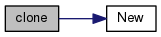## ◆ New()

 Foam::autoPtr< Foam::perfectGas< Specie > > New ( const dictionary & dict )
inlinestatic

Definition at line 60 of file perfectGasI.H.

References dict.

Referenced by perfectGas< Specie >::clone().

Here is the caller graph for this function: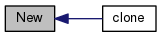## ◆ typeName()

 static word typeName ( )
inlinestatic

Return the instantiated type name.

Definition at line 111 of file perfectGas.H.

## ◆ rho()

 Foam::scalar rho ( scalar p, scalar T ) const
inline

Return density [kg/m^3].

Definition at line 71 of file perfectGasI.H.

References R, and Foam::T().

Here is the call graph for this function: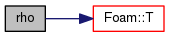## ◆ H()

 Foam::scalar H ( const scalar p, const scalar T ) const
inline

Return enthalpy departure [J/kg].

Definition at line 78 of file perfectGasI.H.

## ◆ Cp()

 Foam::scalar Cp ( scalar p, scalar T ) const
inline

Return Cp departure [J/(kg K].

Definition at line 85 of file perfectGasI.H.

## ◆ S()

 Foam::scalar S ( const scalar p, const scalar T ) const
inline

Return entropy [J/(kg K)].

Definition at line 92 of file perfectGasI.H.

References Foam::log(), Foam::constant::standard::Pstd, and R.

Here is the call graph for this function: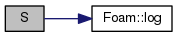## ◆ psi()

 Foam::scalar psi ( scalar p, scalar T ) const
inline

Return compressibility rho/p [s^2/m^2].

Definition at line 99 of file perfectGasI.H.

References R, and Foam::T().

Here is the call graph for this function: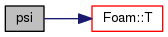## ◆ Z()

 Foam::scalar Z ( scalar p, scalar T ) const
inline

Return compression factor [].

Definition at line 106 of file perfectGasI.H.

## ◆ CpMCv()

 Foam::scalar CpMCv ( scalar p, scalar T ) const
inline

Return (Cp - Cv) [J/(kg K].

Definition at line 113 of file perfectGasI.H.

References R.

## ◆ write()

 void write ( Ostream & os ) const

Write to Ostream.

Definition at line 41 of file perfectGas.C.

References Foam::blockMeshTools::write(), and Ostream::write().

Here is the call graph for this function: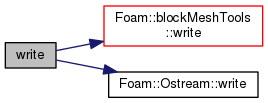## ◆ operator+=()

 void operator+= ( const perfectGas< Specie > & )
inline

Definition at line 122 of file perfectGasI.H.

## ◆ operator*=()

 void operator*= ( const scalar s )
inline

Definition at line 129 of file perfectGasI.H.

References s().

Here is the call graph for this function: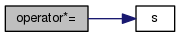## ◆ operator+

 perfectGas operator+ ( const perfectGas< Specie > & , const perfectGas< Specie > & )
friend

## ◆ operator*

 perfectGas operator* ( const scalar s, const perfectGas< Specie > & )
friend

## ◆ operator==

 perfectGas operator== ( const perfectGas< Specie > & , const perfectGas< Specie > & )
friend

## ◆ operator

 Ostream& operator ( Ostream & , const perfectGas< Specie > & )
friend

## ◆ incompressible

 const bool incompressible = false
static

Is the equation of state is incompressible i.e. rho != f(p)

Definition at line 120 of file perfectGas.H.

## ◆ isochoric

 const bool isochoric = false
static

Is the equation of state is isochoric i.e. rho = const.

Definition at line 123 of file perfectGas.H.

The documentation for this class was generated from the following files:
• src/thermophysicalModels/specie/equationOfState/perfectGas/perfectGas.H
• src/thermophysicalModels/specie/equationOfState/perfectGas/perfectGas.C
• src/thermophysicalModels/specie/equationOfState/perfectGas/perfectGasI.H